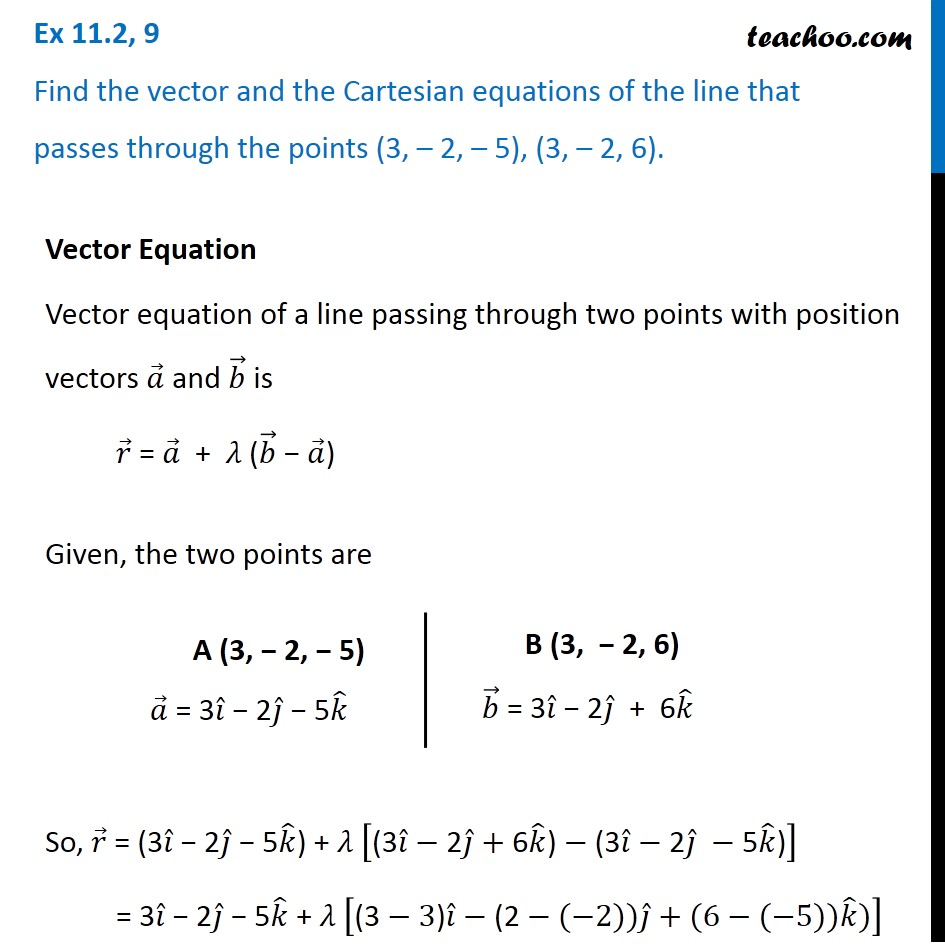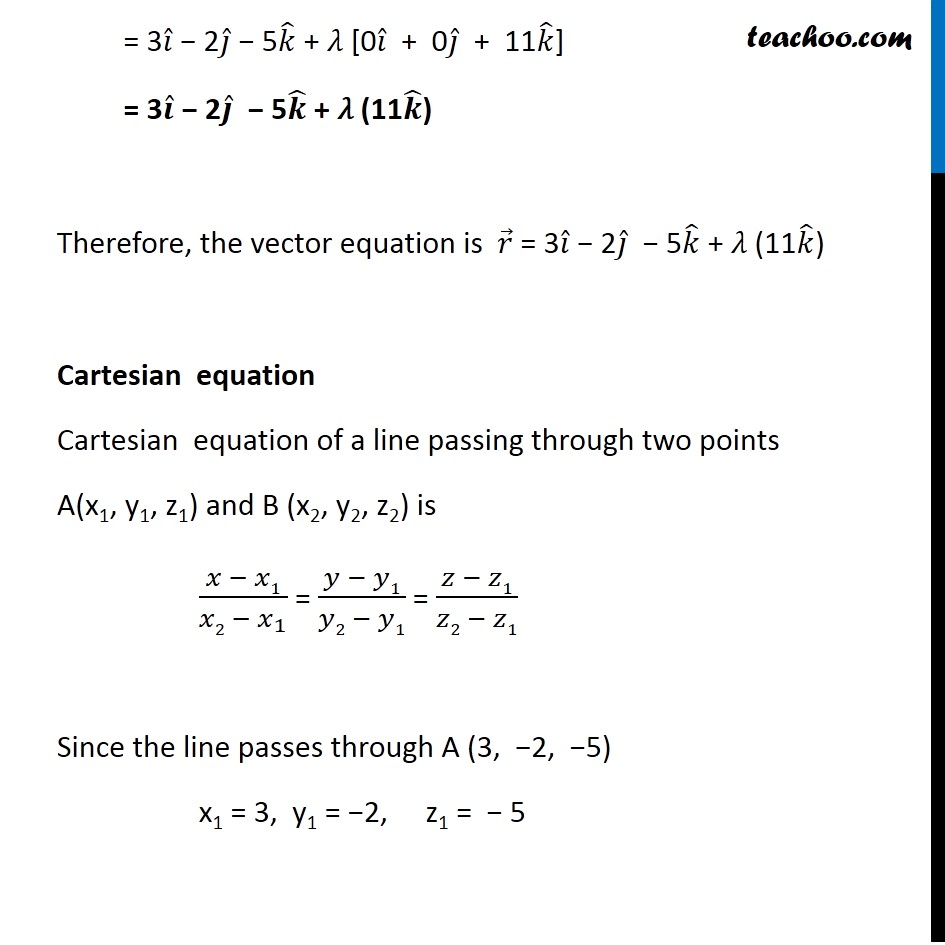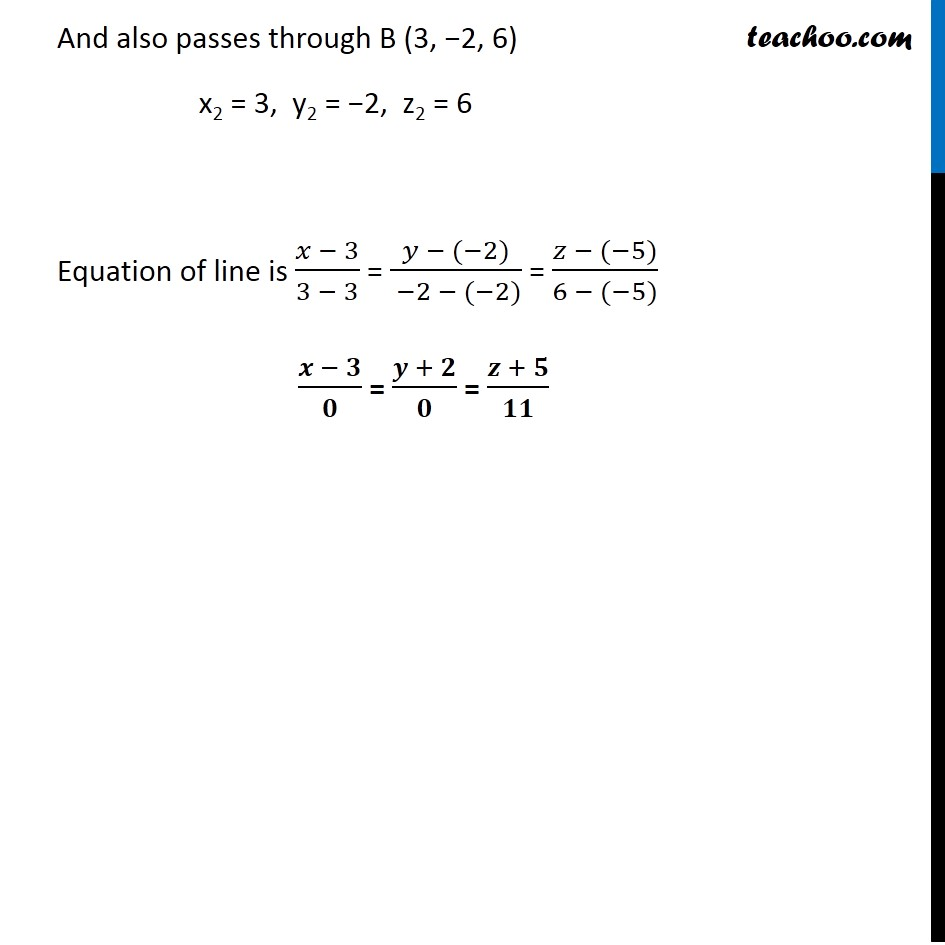Ex 11.2

Chapter 11 Class 12 Three Dimensional Geometry (Term 2)
Serial order wiseNow learn Economics at Teachoo for Class 12

### Transcript

Ex 11.2, 9 Find the vector and the Cartesian equations of the line that passes through the points (3, – 2, – 5), (3, – 2, 6).Vector Equation Vector equation of a line passing through two points with position vectors 𝑎 ⃗ and 𝑏 ⃗ is 𝑟 ⃗ = 𝑎 ⃗ + 𝜆 (𝑏 ⃗ − 𝑎 ⃗) Given, the two points are So, 𝑟 ⃗ = (3𝑖 ̂ − 2𝑗 ̂ − 5𝑘 ̂) + 𝜆 ["(3" 𝑖 ̂−"2" 𝑗 ̂+"6" 𝑘 ̂")" −"(3" 𝑖 ̂−"2" 𝑗 ̂ −"5" 𝑘 ̂")" ] = 3𝑖 ̂ − 2𝑗 ̂ − 5𝑘 ̂ + 𝜆 ["(3" −3")" 𝑖 ̂−"(2" −(−2))𝑗 ̂+(6−(−5))𝑘 ̂)] A (3, − 2, − 5) 𝑎 ⃗ = 3𝑖 ̂ − 2𝑗 ̂ − 5𝑘 ̂ B (3, − 2, 6) 𝑏 ⃗ = 3𝑖 ̂ − 2𝑗 ̂ + 6𝑘 ̂ = 3𝑖 ̂ − 2𝑗 ̂ − 5𝑘 ̂ + 𝜆 [0𝑖 ̂ + 0𝑗 ̂ + 11𝑘 ̂] = 3𝒊 ̂ − 2𝒋 ̂ − 5𝒌 ̂ + 𝜆 (11𝒌 ̂) Therefore, the vector equation is 𝑟 ⃗ = 3𝑖 ̂ − 2𝑗 ̂ − 5𝑘 ̂ + 𝜆 (11𝑘 ̂) Cartesian equation Cartesian equation of a line passing through two points A(x1, y1, z1) and B (x2, y2, z2) is (𝑥 − 𝑥1)/(𝑥2 − 𝑥_1 ) = (𝑦 − 𝑦1)/(𝑦2 − 𝑦1) = (𝑧 − 𝑧1)/(𝑧2 − 𝑧1) Since the line passes through A (3, −2, −5) x1 = 3, y1 = −2, z1 = − 5 And also passes through B (3, −2, 6) x2 = 3, y2 = −2, z2 = 6 Equation of line is (𝑥 − 3)/(3 − 3) = (𝑦 − (−2))/( −2 − (−2)) = (𝑧 − (−5))/(6 − (−5)) (𝒙 − 𝟑)/𝟎 = (𝒚 + 𝟐)/𝟎 = (𝒛 + 𝟓)/𝟏𝟏